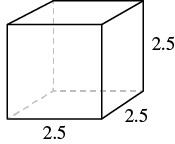## Finding the Volume and Surface Area of a Cube

### Learning Outcomes

• Find the volume and surface area of a cube

A cube is a rectangular solid whose length, width, and height are equal. See Volume and Surface Area of a Cube, below. Substituting, s for the length, width and height into the formulas for volume and surface area of a rectangular solid, we get:

$\begin{array}{ccccc}V=LWH\hfill & & & & S=2LH+2LW+2WH\hfill \\ V=s\cdot s\cdot s\hfill & & & & S=2s\cdot s+2s\cdot s+2s\cdot s\hfill \\ V={s}^{3}\hfill & & & & S=2{s}^{2}+2{s}^{2}+2{s}^{2}\hfill \\ & & & & S=6{s}^{2}\hfill \end{array}$
So for a cube, the formulas for volume and surface area are $V={s}^{3}$ and $S=6{s}^{2}$.

### Volume and Surface Area of a Cube

For any cube with sides of length $s$,### example

A cube is $2.5$ inches on each side. Find its 1. volume and 2. surface area.

Solution
Step 1 is the same for both 1. and 2., so we will show it just once.

 Step 1. Read the problem. Draw the figure and label it with the given information.1. Step 2. Identify what you are looking for. the volume of the cube Step 3. Name. Choose a variable to represent it. let V = volume Step 4. Translate. Write the appropriate formula. $V={s}^{3}$ Step 5. Solve. Substitute and solve. $V={\left(2.5\right)}^{3}$ $V=15.625$ Step 6. Check: Check your work. Step 7. Answer the question. The volume is $15.625$ cubic inches.
 2. Step 2. Identify what you are looking for. the surface area of the cube Step 3. Name. Choose a variable to represent it. let S = surface area Step 4. Translate. Write the appropriate formula. $S=6{s}^{2}$ Step 5. Solve. Substitute and solve. $S=6\cdot {\left(2.5\right)}^{2}$ $S=37.5$ Step 6. Check: The check is left to you. Step 7. Answer the question. The surface area is $37.5$ square inches.

### example

A notepad cube measures $2$ inches on each side. Find its 1. volume and 2. surface area.